# showing real and imaginary numbers in multisim

#### ninjaman

Joined May 18, 2013
324
hello
I want to show the real and imaginary parts of my tuned circuit in mulitsim. I have tried the, simulate>analysis>single AC frequency analysis
I chose the currents through each branch and ended up with this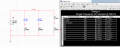this is the result that I get?
the current should be in milliamps, 1.26mA 1.26mA 1.49mA and 4mA.

im not sure what im doing wrong. I have searched around but cant find anything.

any help would be great
I think it may be something to do with expressions. like adding currents or something

thanks
simon

#### crutschow

Joined Mar 14, 2008
25,118
The current in each element in series is the same, as the analysis shows. You don't add them to get total current.
When you add the currents of two elements in series [(such as I(C2) +I(R1)] you get double the current but that is not a real current, just an arbitrary calculation of current that does not appear in the circuit.

#### ninjaman

Joined May 18, 2013
324
the problem is, no of the values that I have on multisim match the values that I have calculated.
I will attach how I worked out the inductor current in complex and polar notation. its not the same and I don't know why.

L= 0.15H
R=4700
F=500Hz
V=7.07VRMS
Inductive reactance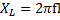=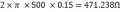Total Impedance using Pythagoras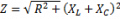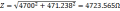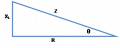To find the current in this branch using Ohms Law: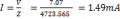Phase angle of current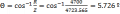The total current is acting at the same phase angle as the impedance above; therefore the current can be expressed in rectangular form(X, Y). X being in the real plane and Y being in the imaginary.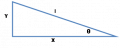Converting to j notation:
Use Trig (or calculator) to derive X and Y quantities.
Using the calculator REC function = Rec (quantity, angle)
Rec (1.49x10-3, 5.726) X=1.483 x10-3
Y=1.487 x10-3
In complex notation, current can be expressed as =1.483x10-3 +j1.487x10-3
Once in complex notation, add all real elements and add all imaginary elements.
These can be put back into calculator (or use trig)
Pol(x,y)
e.g. Pol(3,4) r=5 (total current) –which can be verified on Multisim
θ=53.13º (phase angle)

#### MikeML

Joined Oct 2, 2009
5,444
I dont own Multisim; I use LTSpice.

When you specify a voltage source amplitude, LTSpice takes it as zero to peak value (not rms). It reports the magnitude and phase of the node voltages and branch currents in peak values, not rms. If you specify a voltage source amplitude of {peak/1.414213}, LTSpice carries the reduced voltage through all of the calculations (the circuit has no non-linear components; if it did, AC analysis wouldn't be appropriate, anyway).

Here is a comparison of two circuits. Note the currents. I'll bet Multisim uses the same convention...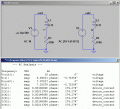#### crutschow

Joined Mar 14, 2008
25,118
Mike, transient AC sources do use peak values, but AC values are all relative to the input so it makes no difference whether they are peak or RMS as long as both input and output values use the same factor.

•MikeML

#### MikeML

Joined Oct 2, 2009
5,444
Isn't that what I tried to say. However, the TS used peak in his sim, but used rms in his hand calcs.

#### crutschow

Joined Mar 14, 2008
25,118
Isn't that what I tried to say. However, the TS used peak in his sim, but used rms in his hand calcs.
Yes. I didn't notice that he converted peak to RMS for his calculations.#### ninjaman

Joined May 18, 2013
324
what is TS?
sorry but that doesn't help. I don't have a lot of time to learn another spice software. I would like to stick to multisim.

thanks for your help, I will leave it though.

simon

#### MikeML

Joined Oct 2, 2009
5,444
what is TS?
sorry but that doesn't help. I don't have a lot of time to learn another spice software. I would like to stick to multisim.

Read the text in my posting. I showed you where you screwed up. Hint, it proves you haven't learned multisim.